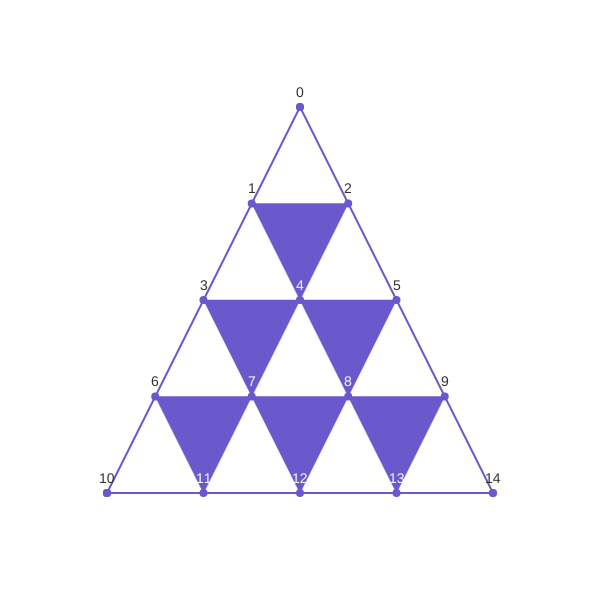## Bézier triangular patches defined as Plotly trisurfs

One year ago we presented here a method for visualizing Bézier triangular surfaces via Python Plotly. Since at that time Plotly could plot only rectangular patches we devised a tricky method to associate a rectangular meshgrid to a triangulation. Now Plotly can plot trisurfs, and here we present how we triangulate the parameter domain of a triangular patch in order to plot it as a trisurf.

The new method is based on the theoretical presentation of Bézier triangular surfaces made in the previous notebook.

In :
import numpy as np
from __future__ import division


Below we define functions that perform the triangulation of the equilateral triangle $\Delta$, in $\mathbb{R}^3$, of vertices $(0,0,1)$, $(1,0,0)$, $(0,1,0)$. Unlike the triangulation method provided by matplotlib.tri, we define a triangulation that returns the barycentric coordinates of vertices, not the cartesian ones.

The triangulate function called for an integer p returns the vertices of a triangulation having $p+1$ points on each side of the triangle $\Delta$, simplices returns the indices corresponding to vertices that form the unfilled triangles in the image below, while simplicesCompl returns the indices corresponding to vertices of filled triangles:

In :
from IPython.display import Image
Image(filename='Imag/triangulgroups.png')

Out:In :
def triangulate(p):
I=range(p, -1, -1)
return [(i/p,j/p, 1-(i+j)/p) for i in I for j in range(p-i, -1, -1)]

def simplices(p):

triplets=[]
i=0
j=1
for nr in range(1,p+1):
for k in range(nr):
triplets.append([i,j,j+1])
i+=1
j+=1
j+=1
return np.array(triplets).reshape((len(triplets), 3))

In :
def simplicesCompl(p):

triplets=[]
i=1
j=2
t=4
m=2
for nr in range(1,p):
for k in range(nr):
triplets.append([i,j,t])
if k<nr-1:
i=i+1
j=j+1
t+=1
i=j+1
j=i+1
t=t+nr+m
m-=1
return np.array(triplets).reshape((len(triplets), 3))

In :
def simplicesAll(p):# a function that returns all simplices returned by both functions above

triplets=[]
i=0
j=1
for nr in range(1,p+1):
for k in range(nr):
triplets.append([i,j,j+1])
i+=1
j+=1
j+=1
i=1
j=2
t=4
m=2
for nr in range(1,p):
for k in range(nr):
triplets.append([i,j,t])
if k<nr-1:
i=i+1
j=j+1
t+=1
i=j+1
j=i+1
t=t+nr+m
m-=1
return np.array(triplets).reshape((len(triplets), 3))


A point of the triangular surface is evaluated according to the triangular de Casteljau algorithm.

In :
def deCasteljau_step(n, b, lam):
#n is the polynomial degree of the surface
# b is the list of control points
#lam is a triplet representing baricentric coordinates of a point in the associated
#triangulation of Delta
i=0
j=1
for nr in range(1, n+1):
for k in range(nr):
b[i]=lam*b[i]+lam*b[j]+lam*b[j+1]
i+=1
j+=1
j+=1
return b[:-(n+1)]

In :
def deCasteljau(n,b,lam):
#recursive function that returns a point on the triangular surface corresponding to
#the barycentric coordinates [lam, lam, lam

if len(b)>1:
return deCasteljau(n-1, deCasteljau_step(n, b, lam), lam)
else:
return b


To each point (triplet of barycentric coordinates) in a triangulation one associates a point on the Bézier patch.

The following function discretizes a Bézier surface of degree n, control points b, and triangulation vertices ( barycenters), defined above:

In :
def surface_points(n, b, barycenters):

points=[]
for weight in barycenters:
b_aux=np.array(b)
points.append(deCasteljau(n, b_aux, weight))
return zip(*points)


set_data_for_Surface prepare data for a plot:

In :
def set_data_for_Surface(n, b, p):

if len(b)!=(n+1)*(n+2)/2:
raise ValueError('incorect number of control points')
barycenters=triangulate(p)

x,y,z=surface_points(n, b, barycenters   )
return x,y, z

In :
def map_z2color(zval, cmap, vmin, vmax):
#map the normalized value zval to a corresponding color in the colormap cmap

if vmin>=vmax:
raise ValueError('incorrect relation between vmin and vmax')
t=(zval-vmin)/float((vmax-vmin))#normalize val
C=map(np.uint8, np.array(cmap(t)[:3])*255)
return 'rgb'+str((C, C, C))

def tri_indices(simplices):
#simplices is a numpy array defining the simplices of the triangulation
#returns the lists of indices i, j, k

return ([triplet[c] for triplet in simplices] for c in range(3))

In :
import plotly.plotly as py
from plotly.graph_objs import *
import matplotlib.cm as cm
import plotly
plotly.offline.init_notebook_mode()